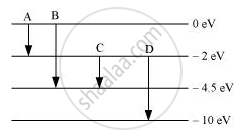Department of Pre-University Education, KarnatakaPUC Karnataka Science Class 12

# The Energy Levels of an Atom Are as Shown Below. Which of Them Will Result in the Transition of a Photon of Wavelength 275 Nm? - Physics

#### Question

The energy levels of an atom are as shown below. Which of them will result in the transition of a photon of wavelength 275 nm?#### Solution

Energy transitions for A,B,C, and D are:

A = 2 eV

B = 4.5 eV

C = 2.5 eV

D = 8 eV

E=(hC)/lamda

Where,

= Energy transition

λ = Wavelength

h = 6.63 × 10−34 Js

C = 3 × 108 m/s

For B, we have

lambda = (6.63 xx 10^-34 xx 3 xx 10^8)/(4.5 xx 1.6 xx 10^-19)

lambda = 275nm

Thus, B will result in transition of a photon of wavelength of 275 nm.

Is there an error in this question or solution?

#### Video TutorialsVIEW ALL 

The Energy Levels of an Atom Are as Shown Below. Which of Them Will Result in the Transition of a Photon of Wavelength 275 Nm? Concept: Energy Levels.